### Home > CALC > Chapter 8 > Lesson 8.1.3 > Problem8-31

8-31.
1. Find h so that the functions below are continuous. Homework Help ✎

1.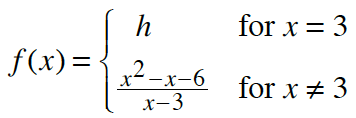2.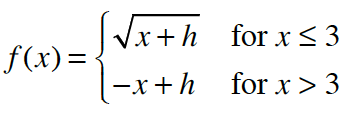3. Determine where the functions in parts (a) and (b) are differentiable.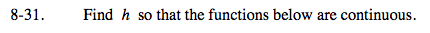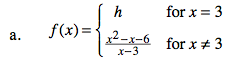After factoring, you will see that the bottom piece has a hole at x = 3. If the f(x) were continuous, then that hole needs to be filled in.
What y-value would fill in the hole?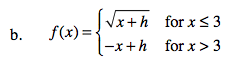Side note: Even after finding the value of h that makes f(x) continuous at x = 3, f(x) will remain discontinuous at x = 0.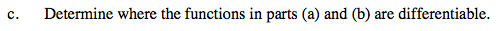Does the derivative exist at the boundary points? In other words, do the slopes agree from the left and the right?